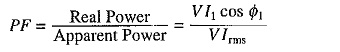## Drawbacks of Rectifier Fed DC Drives:

Drawbacks of Rectifier Fed DC Drives are as follows:

### 1. Distortion of Supply:

Source current of a rectifier has harmonics. In a weak ac source, with high internal impedance, current harmonics distort source voltage. Furthermore, temporary short circuit of lines during commutation of thyristors, causes sharp current pulses, which further distort source voltage. Source voltage and current distortions have several undesirable effects including interference with other loads connected to the source and radio frequency interference in communication equipment.

### 2. Low power factor:

Assuming sinusoidal supply voltage, power factor (PF) of a rectifier can be defined aswhere

V = rms source voltage, V

Irms = rms source current, A

I1 = fundamental component of source current, A

Φ1 = phase difference between V and I1, rad

Thereforewhere μ is called the distortion factor and cos Φ1 is the displacement factor. The distortion in source current makes μ lower than 1. When motor current is assumed to be perfect dc, Φ1 has a value of α for fully controlled single phase and three phase rectifiers and α/2 for single phase half controlled rectifiers, thus giving displacement factors of cos α and cos α/2 respectively. Therefore, supply power factor is low when the drive operates at low speeds.

Pulsewidth modulated rectifiers are being built using insulated gate bipolar transistors (IGBT) and gate turn-off thyristors (GTO) as they have high power factor and low harmonic content in source current but then their efficiency is low because of high switching losses.

### 3. Ripple in Motor Current:

The rectifier output voltage is not perfect dc but consists of harmonics in addition to dc component. Therefore, motor current also has harmonics in addition to dc component. The presence of harmonics, makes rms and peak values of motor currents higher than average value (dc component). Since flux is constant, torque is contributed only by the average value of current. The harmonics produce fluctuating torques, the average value of which is zero.

The presence of harmonics increases both copper loss and core loss. Hence for a allowable temperature rise, the torque and power outputs have lesser values than rated values. Due to the presence of harmonics, peak value of current increases and commutation condition deteriorates. Hence, the current that the motor can commutate without sparking at the brushes has a lower dc component than the rated motor current. Thus the derating of motor occurs due to this also. On the whole the motor output (power and torque) has to be restricted considerably below rated value in order to avoid thermal overloading and sparking at brushes.

Scroll to Top